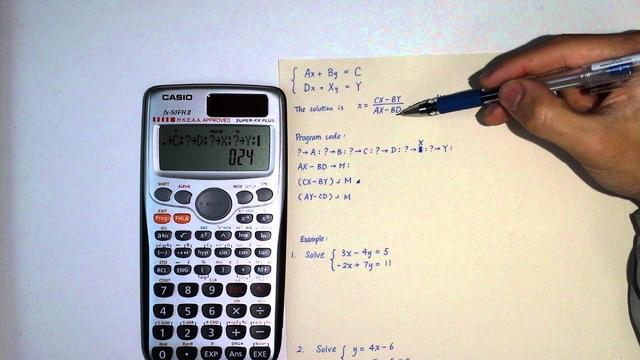# Simultaneous program

## Historical Origins

The book "Nine Chapters of Arithmetic" first listed simultaneous equations in my country and the world. The book "Nine Chapters of Arithmetic" around the AD era, the eighth chapter of "Equations" specifically talks about simultaneous equations. In the equations listed in the book, the unknowns are not represented by symbols, but the coefficients are listed from top to bottom by calculation chips, and the constant items are listed at the bottom to complete a row. For the duality, there are two rows; for the ternary, there are three rows. Since the arrangement of the counting chips is like a square matrix, it is called "equation". The "Equations" chapter introduces the elimination method of simultaneous linear equations. Take the first question of this chapter as an example: "Today there are three upper grains (bundles), two middle grains, one lower grain, and thirty-nine buckets of solid (grain rice); The lower he is one, the actual is thirty-four, the upper he is one, the middle he is two, the lower he is three, and the lower he is twenty-six. How about the upper, middle, and lower hectares?" This is equivalent to modern times. Solve the following ternary linear simultaneous equations:

3x+2y+z=39

2x+3y+z=34

x+2y+3z= 26

Each equation contains three unknowns. Use the principle of elimination to reduce the number of unknowns in the equation to two or one, and the desired result can be obtained. This is essentially the same as the general method in modern algebra.In the 13th century AD, Chinese mathematicians invented a method of formulating equations-Tian Yuan Shu, using the words "Heaven" and "Earth" to represent different unknowns, which can solve binary high-order associations. Cubic formula. The quaternary technique in the "Siyuan Yujian" by Zhu Shijie of the Yuan Dynasty uses the quaternary elements of heaven, earth, man, and matter to express the quaternary higher-order equations. The four-element technique uses the four-element elimination method to solve the problem, which is well-organized.

After the 5th century AD, Indian mathematicians could solve a simultaneous equation. In the West, there was a mathematics book discussing simultaneous equations only after the 16th century. As for the solution of high-order simultaneous equations, it is even more for the future.P>

## ancient Equationh2>

┌────────────────────────────p> < p>│Ⅰ　Ⅱ　Ⅲ　 Today there are three tops, two tops, and one bottom,

│Ⅱ　Ⅲ　Ⅱ There are thirty-nine buckets, two tops, and three tops,

│ Xiahe Yibing, real thirty-four buckets, Shangheyibing,

│Ⅲ　Ⅰ　Ⅰ　 │

│ Zhonghe twobing, bottom He Sanbing, twenty-six fights in reality.

│〓〓〓　 Ask the upper, middle and lower He Shi Yi Bing Ge?

Related Articles